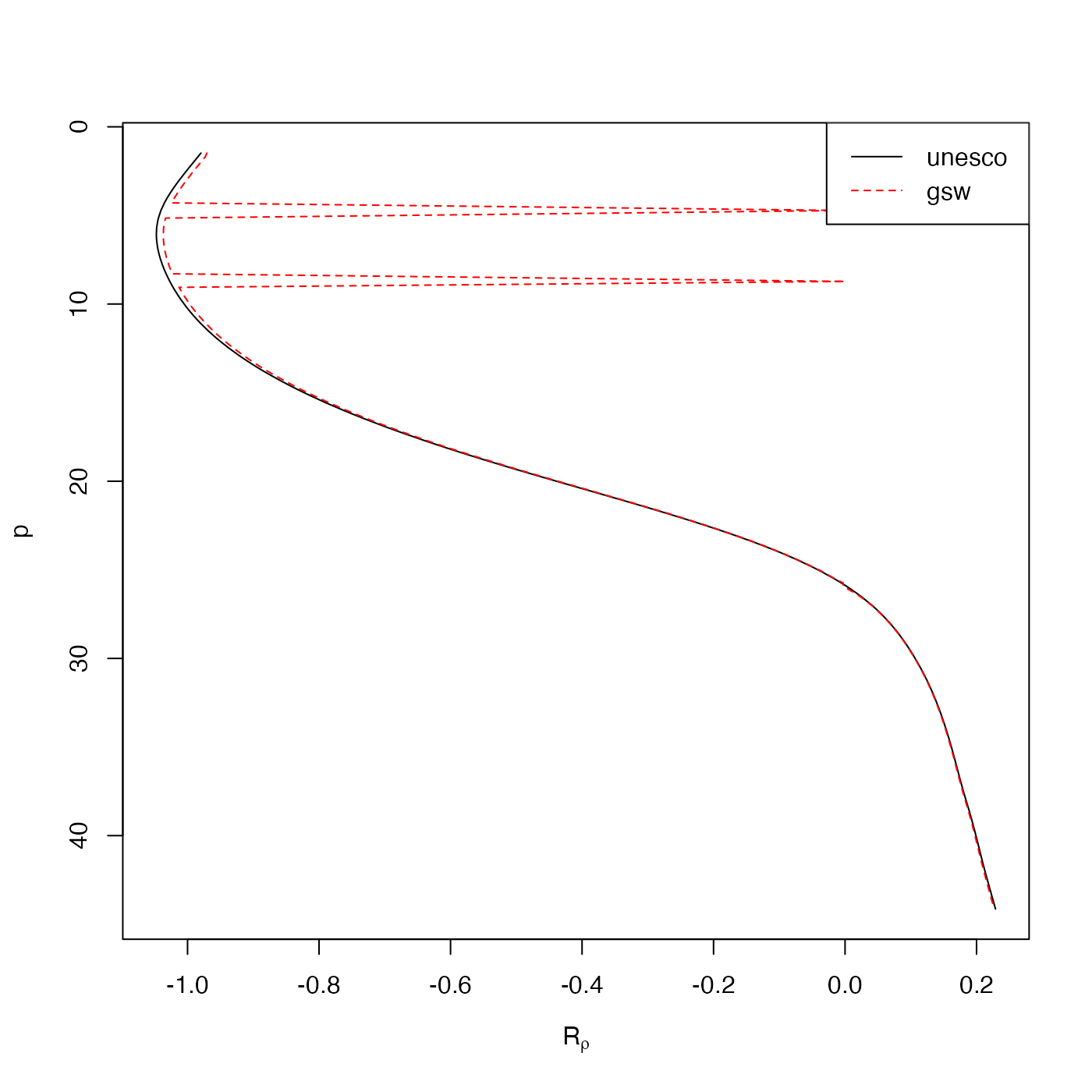Compute density ratio

swRrho(
ctd,
sense = c("diffusive", "finger"),
smoothingLength = 10,
df,
eos = getOption("oceEOS", default = "gsw")
)

## Arguments

ctd

an oce object that holds salinity, temperature, and pressure. If eos is "gsw", then it must also hold longitude and latitude.

sense

an indication of the sense of double diffusion under study and therefore of the definition of Rrho; see “Details”

smoothingLength

ignored if df supplied, but otherwise the latter is calculated as the number of data points, divided by the number within a depth interval of smoothingLength metres.

df

if given, this is provided to smooth.spline().

eos

equation of state, either "unesco" or "gsw".

## Value

Density ratio defined in either the "diffusive" or "finger" sense.

## Details

This computes Rrho (density ratio) from a ctd object.

If eos="unesco", this is done by calculating salinity and potential-temperature derivatives from smoothing splines whose properties are governed by smoothingLength or df. If sense="diffusive" the definition is $$(beta*dS/dz)/(alpha*d(theta)/dz)$$ and the reciprocal for "finger".

If eos="gsw", this is done by extracting absolute salinity and conservative temperature, smoothing with a smoothing spline as in the "unesco" case, and then calling gsw::gsw_Turner_Rsubrho() on these smoothed fields. Since the gsw function works on mid-point pressures, approx() is used to interpolate back to the original pressures.

If the default arguments are acceptable, ctd[["Rrho"]] may be used instead of swRrho(ctd).

Other functions that calculate seawater properties: T68fromT90(), T90fromT48(), T90fromT68(), computableWaterProperties(), locationForGsw(), swAbsoluteSalinity(), swAlphaOverBeta(), swAlpha(), swBeta(), swCSTp(), swConservativeTemperature(), swDepth(), swDynamicHeight(), swLapseRate(), swN2(), swPressure(), swRho(), swSCTp(), swSR(), swSTrho(), swSigma0(), swSigma1(), swSigma2(), swSigma3(), swSigma4(), swSigmaTheta(), swSigmaT(), swSigma(), swSoundAbsorption(), swSoundSpeed(), swSpecificHeat(), swSpice(), swSstar(), swTFreeze(), swTSrho(), swThermalConductivity(), swTheta(), swViscosity(), swZ()

## Author

Dan Kelley and Chantelle Layton

## Examples

library(oce)
data(ctd)
u <- swRrho(ctd, eos="unesco")
g <- swRrho(ctd, eos="gsw")
p <- ctd[["p"]]
plot(u, p, ylim=rev(range(p)), type='l', xlab=expression(R[rho]))
lines(g, p, lty=2, col='red')
legend("topright", lty=1:2, legend=c("unesco", "gsw"), col=c("black", "red"))